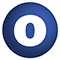# MatrixUtils

#### object MatrixUtils extends Serializable

A collection of utilities useful for matrices.

Linear Supertypes
Serializable, Serializable, AnyRef, Any
Ordering
1. Alphabetic
2. By inheritance
Inherited
1. MatrixUtils
2. Serializable
3. Serializable
4. AnyRef
5. Any
1. Hide All
2. Show all
Visibility
1. Public
2. All

### Value Members

1. #### final def !=(arg0: AnyRef): Boolean

Definition Classes
AnyRef
2. #### final def !=(arg0: Any): Boolean

Definition Classes
Any
3. #### final def ##(): Int

Definition Classes
AnyRef → Any
4. #### final def ==(arg0: AnyRef): Boolean

Definition Classes
AnyRef
5. #### final def ==(arg0: Any): Boolean

Definition Classes
Any

7. #### final def asInstanceOf[T0]: T0

Definition Classes
Any
8. #### def clone(): AnyRef

Attributes
protected[java.lang]
Definition Classes
AnyRef
Annotations
@throws( ... )

10. #### final def eq(arg0: AnyRef): Boolean

Definition Classes
AnyRef
11. #### def equals(arg0: Any): Boolean

Definition Classes
AnyRef → Any
12. #### def finalize(): Unit

Attributes
protected[java.lang]
Definition Classes
AnyRef
Annotations
@throws( classOf[java.lang.Throwable] )
13. #### final def getClass(): Class[_]

Definition Classes
AnyRef → Any
14. #### def hashCode(): Int

Definition Classes
AnyRef → Any
15. #### final def isInstanceOf[T0]: Boolean

Definition Classes
Any
16. #### def isSorted[T](s: Seq[T])(implicit cmp: Ordering[T]): Boolean

Check if a given sequence is sorted.

Check if a given sequence is sorted.

s

Sequence that needs to be checked.

returns

true if the squence is sorted. false otherwise

17. #### def matrixToColArray[T](mat: DenseMatrix[T])(implicit arg0: ClassTag[T]): Array[DenseVector[T]]

Converts a matrix to an array of columns.

Converts a matrix to an array of columns.

mat

Input matrix.

returns

Array of columns.

18. #### def matrixToRowArray[T](mat: DenseMatrix[T])(implicit arg0: ClassTag[T]): Array[DenseVector[T]]

Converts a matrix to an array of rows.

Converts a matrix to an array of rows.

mat

Input matrix.

returns

Array of rows.

19. #### final def ne(arg0: AnyRef): Boolean

Definition Classes
AnyRef
20. #### final def notify(): Unit

Definition Classes
AnyRef
21. #### final def notifyAll(): Unit

Definition Classes
AnyRef
22. #### def rowsToMatrix[T](in: RDD[DenseVector[T]])(implicit arg0: ClassTag[T]): RDD[DenseMatrix[T]]

Converts a RDD of DenseVector to a RDD of DenseMatrix such that vectors in each partition are stacked into a rows to make a DenseMatrix.

Converts a RDD of DenseVector to a RDD of DenseMatrix such that vectors in each partition are stacked into a rows to make a DenseMatrix.

in

RDD of DenseVectors (row)

returns

RDD containing dense matrices

23. #### def rowsToMatrix[T](inArr: Array[DenseVector[T]])(implicit arg0: ClassTag[T]): DenseMatrix[T]

Converts an array of DenseVector to a matrix where each vector is a row.

Converts an array of DenseVector to a matrix where each vector is a row.

inArr

Array of DenseVectors (rows)

returns

A row matrix.

24. #### def rowsToMatrix[T](in: TraversableOnce[DenseVector[T]])(implicit arg0: ClassTag[T]): DenseMatrix[T]

Converts a sequence of DenseVector to a matrix where each vector is a row.

Converts a sequence of DenseVector to a matrix where each vector is a row.

in

Sequence of of DenseVectors (rows)

returns

A row matrix.

25. #### def rowsToMatrixIter[T](in: TraversableOnce[DenseVector[T]])(implicit arg0: ClassTag[T]): Iterator[DenseMatrix[T]]

Converts a sequence of DenseVector to a matrix where each vector is a row.

Converts a sequence of DenseVector to a matrix where each vector is a row.

in

Sequence of of DenseVectors (rows)

returns

Iterator with a single element if rows is non-empty. Empty iterator otherwise.

26. #### def sampleRows(in: DenseMatrix[Double], numSamples: Int): DenseMatrix[Double]

Draw samples rows from a matrix.

Draw samples rows from a matrix.

in

Input matrix.

numSamples

Number of samples to draw.

returns

A matrix constructed from a sample of the rows.

28. #### final def synchronized[T0](arg0: ⇒ T0): T0

Definition Classes
AnyRef
29. #### def toString(): String

Definition Classes
AnyRef → Any

31. #### final def wait(): Unit

Definition Classes
AnyRef
Annotations
@throws( ... )
32. #### final def wait(arg0: Long, arg1: Int): Unit

Definition Classes
AnyRef
Annotations
@throws( ... )
33. #### final def wait(arg0: Long): Unit

Definition Classes
AnyRef
Annotations
@throws( ... )